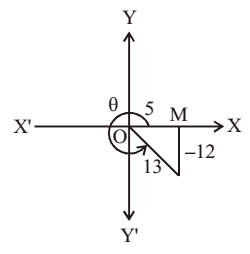## NCERT Solutions for Class 11 Maths Chapter 3 Trigonometric Functions Exercise 3.2

If you're in search of Chapter 3 Trigonometric Functions Exercise 3.2 then you can find here. It will help in completing home task in no time. Practicing the answers will help you improving problem solving skills of students and increase their speed. NCERT Solutions for Class 11 Maths prepared by Studyrankers will make you aware of how to apply formulas.1. Find the value of other five trigonometric function when cos x = -1/2, x lies in third quadrant.

Since x lies in the 3rd quadrant
Cos x = -1/2
∴ Sin x = - √(1 – cos2x) (∵ x lies in III rd  quadrant)
=- √(1 – ¼) = -√3/2
Tan x = √3
Cot x = 1/√3
Sec x = (1/cos x) = -2
Cosec x = 1/sin x = - 2/√3

2. Find the value of other five trigonometric function when  sin x=3/ 5, x lies in second quadrant.

Since x lies in the second quadrant
sin x = 3/5 given
cos x = - √(1 – sin2 x) (∵ x lies in II quadrant)
= - √(1 – 9/25) = -4/5
Sec x = -5/4, tan x = - ¾
Cosec x = 5/3, cot x = - 4/3

3. cot x = 3/4, x lies in third quadrant.

since x lies in third quadrant.∴ cot x = 3/4 = -3/-4
Let MP = -4, OM = -3, then
OP = √(MP2 + OM2) = √(16 + 9)
= √25 = 5
Now sin x = MP/OP = -4/5
cot x = OM/MP = 3/4
cos x = OM/OP = -3/5
sec x = OP/OM = 5/-3 = -5/3
tan x = 4/3 [Given]
cosec x = OP/MP = 5/-4 = -5/4

4. sec x = 13/5, x lies in fourth quadrant.

Since x lies in fourth quadrant.∴ sec x = 13/5 => OP/OM = 13/5
Let OP = 13, OM = 5. Then,
MP = -√(OP2 - OM2)
= - √(169 - 25)
- √144 = -12
Now, sin x = MP/OP = -12/13
cot x = OM/MP = 5/12 = -5/12
cos x = OM/OP = 5/3
sec x = OP/OM = 13/5
tan x = MP/OM = -12/5
cosec x = OP/MP = 13/-12 = -13/12

5. Find the value of other five trigonometric function when tan x = - 5/12, x lies in second quadrant.

x lies in the second quadrant
tan x = -5/12
cot x = - 12/5
sec x = - √(1 + tan2x) ( ∵ x lies in II quadrant)
= - √(1 + 25/144) = -13/12
cos x = -12/13
sin x =  √1- cos2x = 5/13
cosec x = 13/5

6. Find the values of the following trigonometric sin 765°.

sin 765° = sin (8 × 90° + 45°)
= sin 45° 1/√2

7. Find the values of the following trigonometric cosec (– 1410°).

cosec (–1410°)
= cosex(-1410 + 1440) = cosec(30) = 2

8. Find the values of the following trigonometric tan 19π/3.

tan 19π/3 = tan (6 π + π/3) = tan π/3 = √3

9. Find the values of the following trigonometric 11 sin (-11 π/3)